# accounting odd question E 9

During 2007 Federal Express reported the following information (in millions): net sales of \$35,214 and net income of \$2,016. Its balance sheet also showed total assetsat the beginning of the year of \$22,690 and total assets at the end of the year of \$24,000.

Calculate the (a) asset turnover ratio and (b) return on assets ratio.

According to the given problem,Net sales = \$35,214Net income = \$2,016Beginning total assets = \$22,690Ending total assets = \$24,000a) Asset turnover ratio: The formula for calculating the total asset turnover ratio isTotal assetturnover ratio = Net sales / Total assetsBut Total assets value is computed by taking the average of the beginning and ending total assets for the period:Total assets =(Beginning total assets + Ending total assets) / 2= (\$22,690 + \$24,000) / 2= \$23,345Substituting the values in the above formula, we getTotal asset turnoverratio = \$35,214 / \$23,345=1.51 timesTherefore, the total asset turnover ratio is 1.51 timesb) The formula for calculating the Return on total assets isROA = Net income / Total assetsThe total assets value is taken which we calculated for the previous ratioROA= \$2,016 / \$23,345=0.086 or 8.6%Therefore, the return on assets is 8.6%

#### Earn Coin

Coins can be redeemed for fabulous gifts.

Similar Homework Help Questions
• ### Suppose during 2022 that Federal Express reported the following information (in millions): net sales of \$34,750...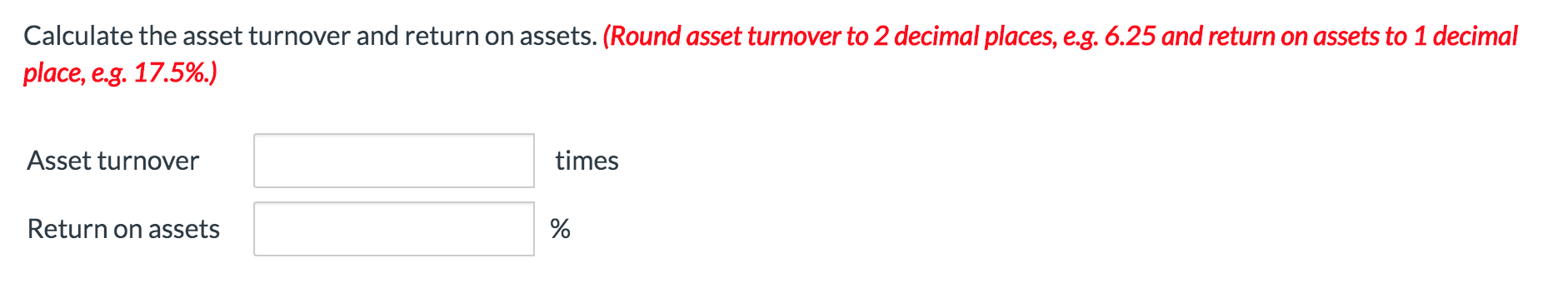Suppose during 2022 that Federal Express reported the following information (in millions): net sales of \$34,750 and net income of \$95. Its balance sheet also showed total assets at the beginning of the year of \$24,650 and total assets at the end of the year of \$23,300. Calculate the asset turnover and return on assets. (Round asset turnover to 2 decimal places, e.g. 6.25 and return on assets to 1 decimal place, e.g. 17.5%.) Asset turnover times Return on assets

• ### – Your answer is partially correct. Suppose during 2022 that Federal Express reported the following information...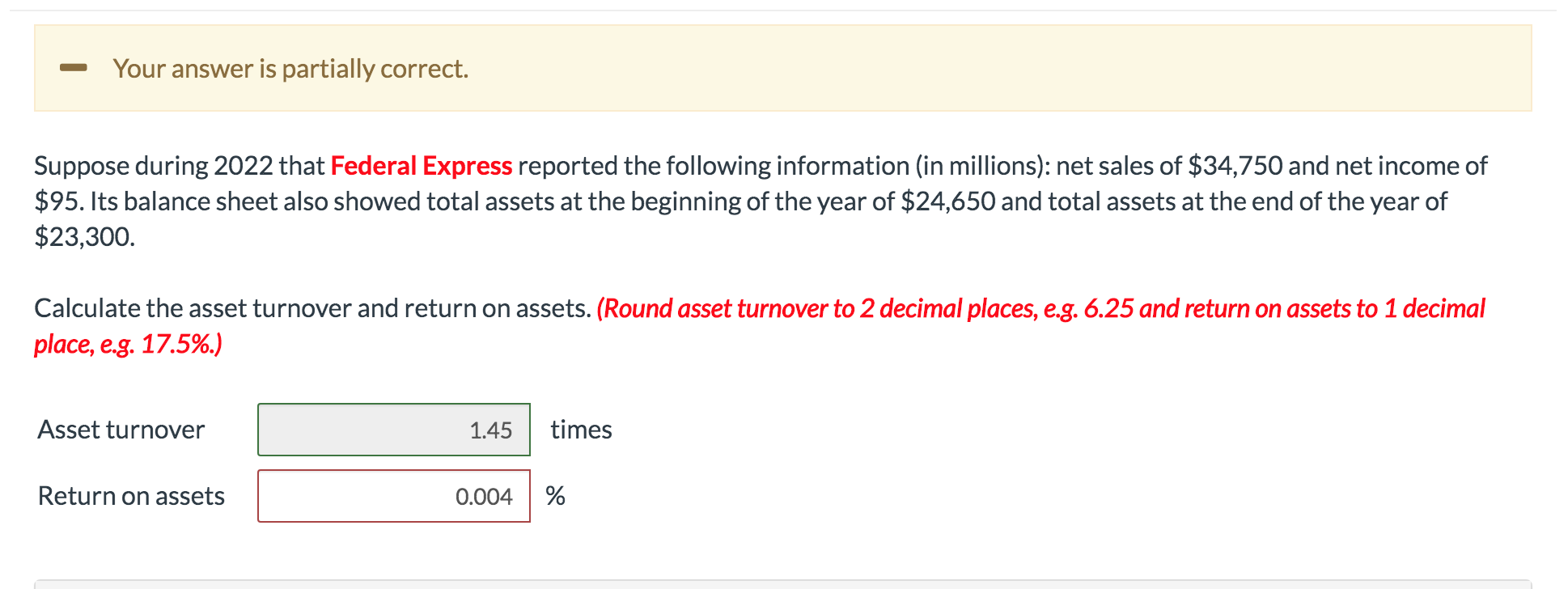– Your answer is partially correct. Suppose during 2022 that Federal Express reported the following information (in millions): net sales of \$34,750 and net income of \$95. Its balance sheet also showed total assets at the beginning of the year of \$24,650 and total assets at the end of the year of \$23,300. Calculate the asset turnover and return on assets. (Round asset turnover to 2 decimal places, e.g. 6.25 and return on assets to 1 decimal place, e.g. 17.5%.)...

• ### Send to Gradebook Question 7 View Policies Current Attempt in Progress Suppose during 2022 that Federal...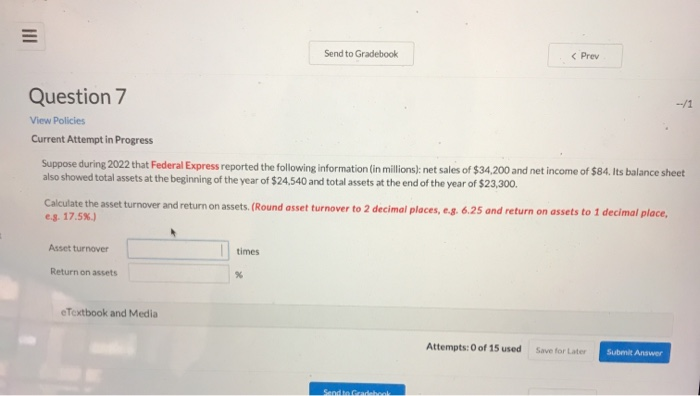Send to Gradebook Question 7 View Policies Current Attempt in Progress Suppose during 2022 that Federal Express reported the following information (in millions): net sales of \$34,200 and net income of \$84. Its balance sheet also showed total assets at the beginning of the year of \$24,540 and total assets at the end of the year of \$23,300. Calculate the asset turnover and return on assets. (Round asset turnover to 2 decimal places, .s. 6.25 and return on assets to...

• ### Question A Question B Question C Question E Sheridan Company purchased a new machine on October...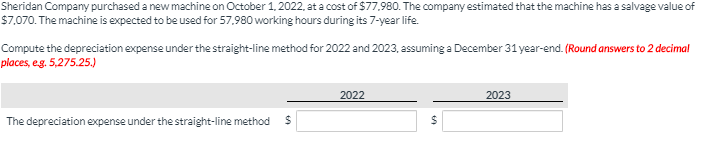Question A Question B Question C Question E Sheridan Company purchased a new machine on October 1, 2022. at a cost of \$77.980. The company estimated that the machine has a salvage value of \$7.070. The machine is expected to be used for 57.980 working hours during its 7-year life. Compute the depreciation expense under the straight-line method for 2022 and 2023, assuming a December 31 year-end. (Round answers to 2 decimal places, eg. 5,275.25.) 2022 - 2023 The depreciation...

• ### Cadux Candy Company's income statement for the year ended December 31, 2021, reported interest expense of...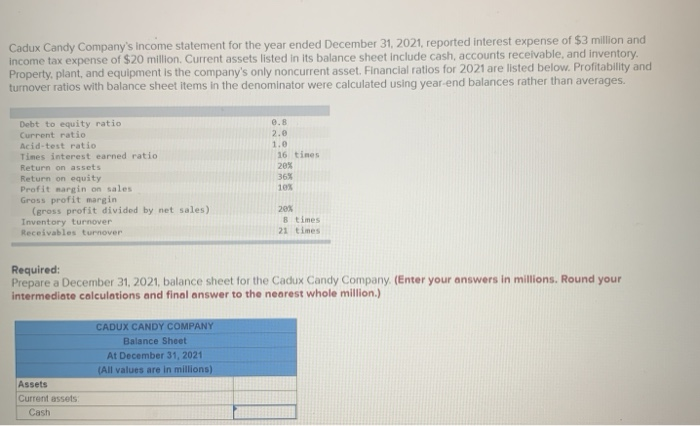Cadux Candy Company's income statement for the year ended December 31, 2021, reported interest expense of \$3 million and income tax expense of \$20 million. Current assets listed in its balance sheet include cash, accounts receivable, and inventory. Property, plant, and equipment is the company's only noncurrent asset. Financial ratios for 2021 are listed below. Profitability and turnover ratios with balance sheet items in the denominator were calculated using year-end balances rather than averages. Debt to equity ratio Current ratio...

• ### Please use the following financial data to answer questions (a) through (e) regarding Marquez Corp

12. Please use the following financial data to answer questions (a) through (e) regarding Marquez Corp. (15 points)Marquez Balance SheetDecember 31, 2007AssetsCurrent Assets \$100,000Plant Assets 150,000Total Assets 250,000 =======Liabilities and shareholders’ equityCurrent Liabilities \$100,000Long-Term Liabilities 75,000Total Liabilities \$175,000Stockholders’ Equity 75,000Total Liabilities & Stockholders’ Equity \$250,000 ========Income Statement data:Net Sales \$375,000Interest Expense 4,000Net Income 22,500The following account balances existed at December 31, 2007Total Assets \$200,000Stockholders’ Equity 65,000The tax rate is 35%. Industry Norms as of December 31, 2007 are:Debt/Equity Ratio 1.75Profit...

• ### Please show me how to calculate this problem: During 2007, Sitter Corporation reported net sales of \$2,000,000, net income of \$1,200,000, and depreciation expense of \$100,000

Please show me how to calculate this problem: During 2007, Sitter Corporation reported net sales of \$2,000,000, net income of \$1,200,000, and depreciation expense of \$100,000. Sitter also beginning total asset of \$1,000,000, ending total asset of \$1,500,000, plant asset of \$800,000, and accumulated depreciation of \$500,000. Sitter’s asset turnover ratio is:

• ### Cadux Candy Company's income statement for the year ended December 31, 2021, reported interest expense of...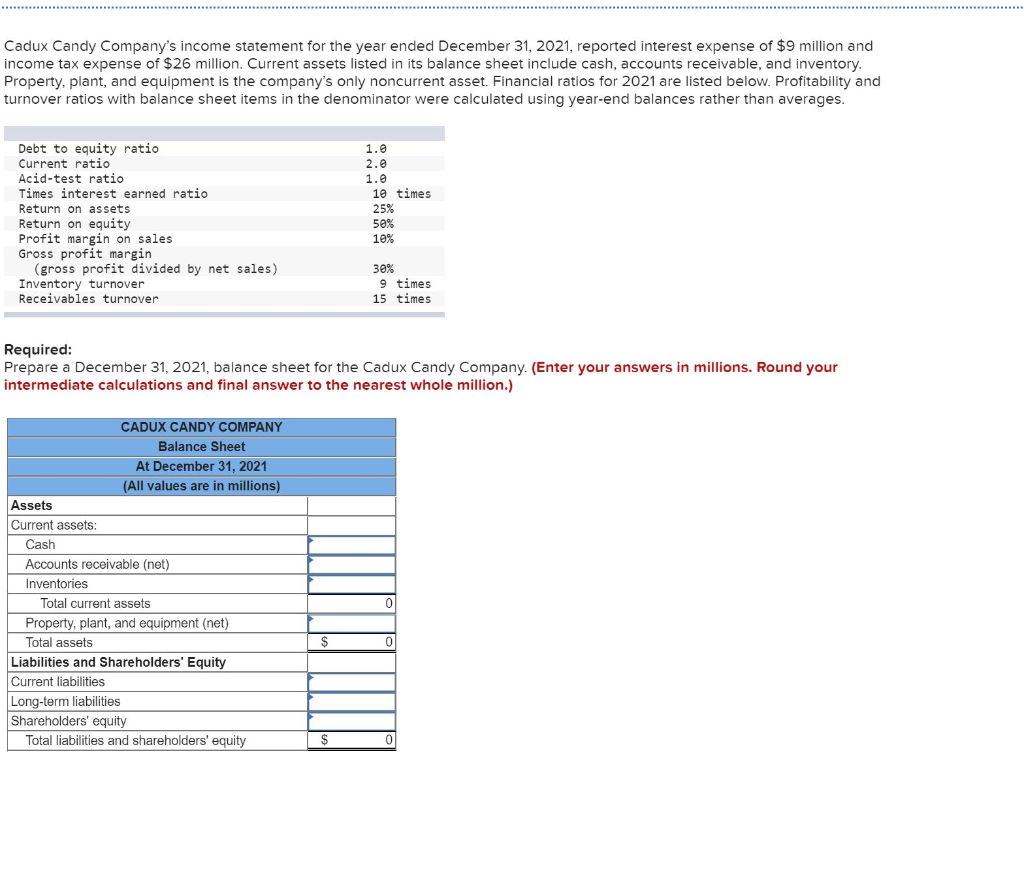Cadux Candy Company's income statement for the year ended December 31, 2021, reported interest expense of \$9 million and income tax expense of \$26 million. Current assets listed in its balance sheet include cash, accounts receivable, and inventory. Property, plant, and equipment is the company's only noncurrent asset. Financial ratios for 2021 are listed below. Profitability and turnover ratios with balance sheet items in the denominator were calculated using year-end balances rather than averages. Debt to equity ratio Current ratio...

• ### Brad's BBQ reported sales of \$780,000 and net income of \$32,500. Brad’s also reported ending total...

Brad's BBQ reported sales of \$780,000 and net income of \$32,500. Brad’s also reported ending total assets of \$499,000 and beginning total assets of \$392,000. Required: Calculate the return on assets, the profit margin, and the asset turnover ratio for Brad's BBQ.

• ### Brad's BBQ reported sales of \$810,000 and net income of \$35,500. Brad's also reported ending total...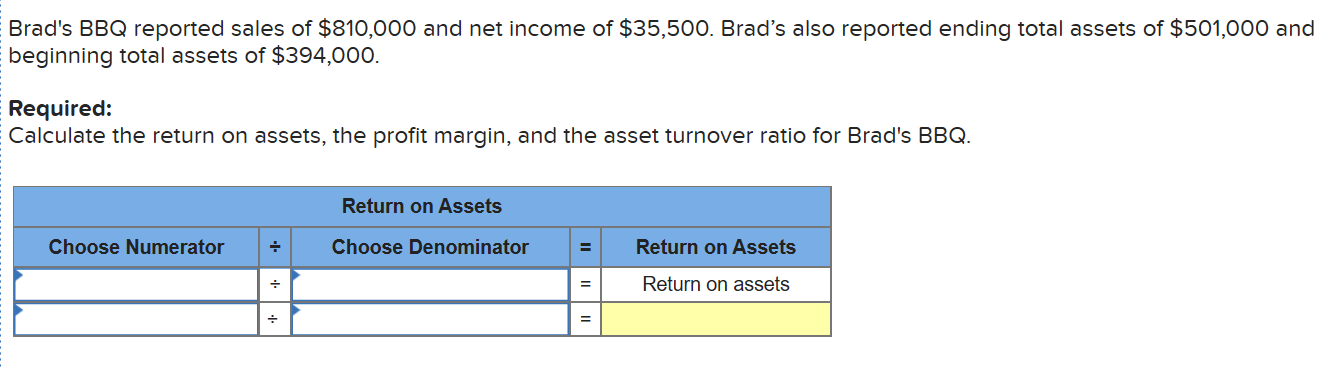Brad's BBQ reported sales of \$810,000 and net income of \$35,500. Brad's also reported ending total assets of \$501,000 and beginning total assets of \$394,000. Required: Calculate the return on assets, the profit margin, and the asset turnover ratio for Brad's BBQ. Return on Assets Choose Numerator - Choose Denominator Return on Assets Return on assets .